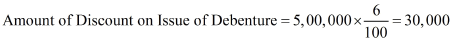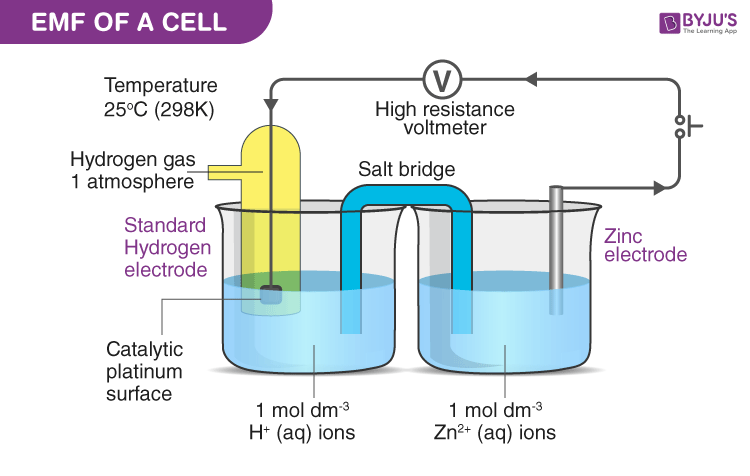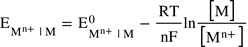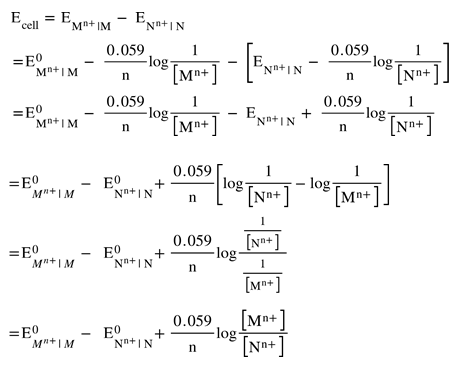# EMF of a Cell

The electromotive force of a cell or EMF of a cell is the maximum potential difference between two electrodes of a cell. It can also be defined as the net voltage between the oxidation and reduction half-reactions. The EMF of a cell is mainly used to determine whether an electrochemical cell is galvanic or not.

## What is an Electrochemical Cell?

An electrochemical cell is a device that generates electricity from a chemical reaction. Essentially, it can be defined as a device that converts chemical energy into electrical energy. A chemical reaction that involves the exchange of electrons is required for an electrochemical cell to operate. Such reactions are called redox reactions.

A cell is characterized by its voltage. A particular kind of cell generates the same voltage irrespective of the size of the cell. The only thing that depends on the cell voltage is the chemical composition of the cell, given the cell is operated at ideal conditions.

Normally, the cell voltage may be different from this ideal value, due to several factors like temperature difference, change in concentration, etc. Nernst equation formulated by Walther Nernst can be used to calculate the EMF value of a given cell, provided the standard cell potential of the cell.

## Types of Electrochemical Cell

### Galvanic Cell

Galvanic Cell is named after Luigi Galvani an Italian scientist. A galvanic cell is an important electrochemical cell that forms the base of many other electrochemical cells like the Daniell cell. It constitutes of two different metallic conductors called electrodes immersed in their own ionic solutions. Each of these arrangements is a half cell. Alone, a half cell is not able to generate a potential difference. But combined, they generate a potential difference. A salt bridge is used to combine the two cells chemically. It serves the required amount of electrons to the electron-deficient half cell and accepts electrons from the electron-rich half cell.

For ease of understanding, let’s look at the theory of the Daniell cell and derive the Nernst equation for the same.

### Daniell Cell

Daniell cell is an adaptation of the galvanic cell. It is constituted of zinc and copper electrodes immersed in zinc sulfate and copper sulfate solutions respectively. Two half cells are connected together using a salt bridge. The zinc electrode as anode and copper act as cathode.

The zinc metal is top in the electrochemical series when comparing to the copper metal, owing to the higher value of the oxidation potential of the metal. Hence, zinc undergoes oxidation, consequently, two electrons and a zinc ion are generated. This electrode acquires a negative potential due to the release of electrons when comparing to the other electrode. We call it an anode.

However, copper undergoes reduction, owing to its higher reduction potential. The copper ion in the solution of the copper half cell accepts two electrons from the electrode and becomes copper metal and gets deposited in the electrode. As this electrode uses up electrons, we consider this electrode as a positive electrode, and we call it cathode.

Anode reaction is represented as follows:

Zn(s) → Zn2+ (aq) + 2e

The cathode reaction is represented as follows:

Cu2+ (aq) +2e → Cu(s)

The combined cell reaction or overall cell reaction is as follows:

Zn(s) + Cu2+(aq) → Zn2+ (aq) + Cu(s)## Electrode Potential

When a metal electrode is immersed in a solution containing its own ions, a potential difference is set up across the interface. This potential difference is called the electrode potential.

Consider the case of the zinc electrode immersed in a zinc sulfate solution. The zinc metal gets oxidized by releasing two electrons and becomes and is dissipated in the solution. The presence of electrons in the electrode and ions in the solution creates a potential difference. Same way, copper develops a positive potential. The combination of these two cells owing to the cell potential.

In reality, we are not able to determine the potential of a single half cell alone. To determine the potential of a single half cell, we always need a standard half cell whose potential value is already known. This standard half cell is then connected with the unknown half cell to determine the overall potential.

This overall potential is the difference between the potentials of the two half cells. The standard hydrogen electrode (SHE) is an example of such a standard half cell. The potential value of SHE is inherently set to zero volts. The standard hydrogen electrode is connected with an unknown half cell and the potential difference is measured. As SHE has zero volts, the measured value will be the potential difference of the unknown half cell.

The picture below represents the method to find the standard electrode potential of zinc.## Electrochemical Series

Likewise, the standard potential values of different metals are calculated and arranged in the increasing order of the potential, we obtain the electrochemical series.

Electrochemical series are essential for the determination of cell potential. It also helps in selecting electrode metals for the construction of a cell.

The electrochemical series table shows the arrangement of a few elements based on the increasing order of their reduction potential. Lithium usually has the least reduction potential and fluorine has the most. Hydrogen has a zero reduction potential. This is because all other elements are compared against hydrogen to obtain their standard electrode potential.

## Representation of an Electrochemical Cell

An electrochemical cell can be represented using special notations. This is useful in understanding the composition as well as it’s quantity in the cell.

The above-given Daniell cell can be represented as follows;

Zn | Zn2+ (1M) || Cu2+ (1M) | Cu

Let us break down this and understand its components:

● The left side of the notation represents the anode. At the anode, Zn is converted to by releasing a set of two electrons per zinc atom. As the solution used is of 1M concentration, we include that too in the representation

Zn | Zn2+ (1M)

● On the right side, we have the cathode. Here from the electrolyte absorbs a pair of electrons from the electrode and gets converted to Cu metal. Same as before, we are using a 1M copper sulfate solution.

Cu2+ (1M) | Cu

● These two half-cells are combined using a salt bridge. The salt bridge is represented using the two vertical bars.

Zn | Zn2+ (1M) || Cu2+ (1M) | Cu

## Finding the Cell Potential of an Electrochemical Cell

The cell potential or EMF of the electrochemical cell can be calculated by taking the values of electrode potentials of the two half – cells. There are usually three methods that can be used for the calculation:

• By taking into account the oxidation potential of anode and reduction potential of cathode.
• By considering the reduction potentials of both electrodes.
• By taking the oxidation potentials of both electrodes.

The standard cell potential (ΔEo) of a galvanic cell can be calculated by considering the standard reduction potentials of the two half cells Eo.

Eocell = Eored + Eoox

Or

Eocell = EoCathode + EoAnode

The cell potential of a galvanic cell = Potential of the half cell on the right side (cathode) – Potential of the half cell on the left (anode).

In the case of Daniell cell;

The cell potential of Daniell cell = Ecell = Ecu2+ | Cu – Ezn | Zn2+

Nernst equation relates the equilibrium state potential of a half cell with the standard electrode potential of the half cell, temperature, reaction coefficients as well as activity of the reacting species.

For an electrode reaction Mn+ + n e → M(s);

The equilibrium state EMF is given by;Where EMn+| M is the half cell potential, EOMn+| M is the standard electrode potential of the half cell, [ M ] is the molar concentration of the metal, [ Mn+ ] is the molar concentration of the metal ion, R is the universal gas constant (8.314 J/K/mole), T is the temperature in kelvin, n is the number of electrons involved in the reaction and F is the Faraday’s constant (96500 C/mole).

The molar concentration of metallic solid is conventionally taken as one. Hence;

[ M ] = 1

Now, substitute the values of R, F, T = 298K (room temperature) to the above equation and we obtain a new equation;

EMn+| M = , EOMn+| M – 0.059 / n X log 1 / [ Mn+ ]

Let us consider an arbitrary cell having two metals M and N. Also let us assume that M has more reduction potential than N. Hence, M forms the cathode and N forms the anode.

Hence, Anode reaction;

N → Nn+ + ne

Cathode reaction;

Mn+ + ne → M

We know;

The cell potential of a galvanic cell = Potential of the half cell on the right side(cathode) – Potential of the half cell on the left(anode).

EMF of cathode half cell;

EMn+| M = , EOMn+| M – 0.059 / n X log 1 / [ Mn+ ]

EMF of anode half cell;

ENn+| N = , EONn+| N – 0.059 / n X log 1 / [ Nn+ ]

Hence, the cell potential of the Galvanic cell is;We have;

EOMn+| M – EONn+| N = EOcell

This is the standard cell potential of a galvanic cell at STP conditions.

Hence we rewrite this as;

Ecell = EOcell + 0.059 / n X log [ Mn+ ] / [ Nn+ ]

For the Daniell cell:

The number of electrons involved in the process is 2, hence n = 2.

Ecell = EOcell + 0.059 / 2 X log [ Cu2+ ] / [ Zn2+ ]

From the electrochemical series;

EOcell = EOcathode – EOanode = ECu2+| Cu = , EOZn2+| Zn = 0.34 – (-0.76) = 1.1 V

Substitute this value and we get;

Ecell = 1.1 + 0.059 / 2 X log [ Cu2+ ] / [ Zn2+ ]

## Solved Examples

1. Calculate the EMF of the below redox reaction.

Mg (s) + 2H+ (aq) → Mg2+ (aq) + H(g)

Solution:

Step 1: Break the reaction into oxidation and reduction half cell reaction

2H+ + 2e → H2

Hydrogen ion takes an electron to form hydrogen gas (reduction reaction).

Magnesium undergo oxidation by loosing two electrons

Mg → Mg2+ + 2 e

Step 2: Find the standard reduction potential of the half-cell reaction

E(Reduction) = 0.0000 V

E(Oxidation) = -2.372 V

E(Oxidation) = – E(Reduction)

= +2.372 V

Step 3: Calculate the total EMF of the cell

E0 (Cell) = E(Reduction) + E(Oxidation)

E0 (Cell) =  0.0000 V + 2.372 V

E0 (Cell) = 2.372 V

The EMF of the redox reaction is +2.372 volts.

2. Calculate the potential of the following redox reaction?

Ag|Ag+||Li+|Li

Solution:

Step 1: Break the reaction into oxidation and reduction half cell reaction

Li+ + e → Li (reduction reaction)

Ag → Ag+ + e– (Oxidation reaction)

Step 2: Find the standard reduction potential of the half-cell reaction

E(Reduction) = -3.045 V

E(Oxidation) = 0.799 V

Step 3: Calculate the total EMF of the cell

E0 (Cell) = E(Reduction) + E(Oxidation)

E0 (Cell) =  -3.045 V 0.799 V

E0 (Cell) = -3.844 V

The total potential of the cell is -3.844 V and the negative potential inndicates the sponteneous reverse reaction.

3. Consider a redox reaction

2Ag+ Cd → 2Ag + Cd2+

The satndard electrode potential of reduction and oxidation reactions are + 0.80 V and -0.40 V  respectively. Calculate the standard potentiol for the reaction.

Solution:

Step 1: Consider a half cell reactions

2Ag+ 2e → 2Ag (Reduction)

EoAg+/Ag =0.80  V

Cd → Cd2+  + 2e (Oxidation)

EoCd+/Cd = -0.40 V

Step 2: Total EMF of the cell

E= E (Reduction) + E (Oxidation)

E= 0.40 V + +0.80 V

E= 1.20 V

The total EMF of the redox reaction is 1.20 V.

Test your Knowledge on emf of a cell